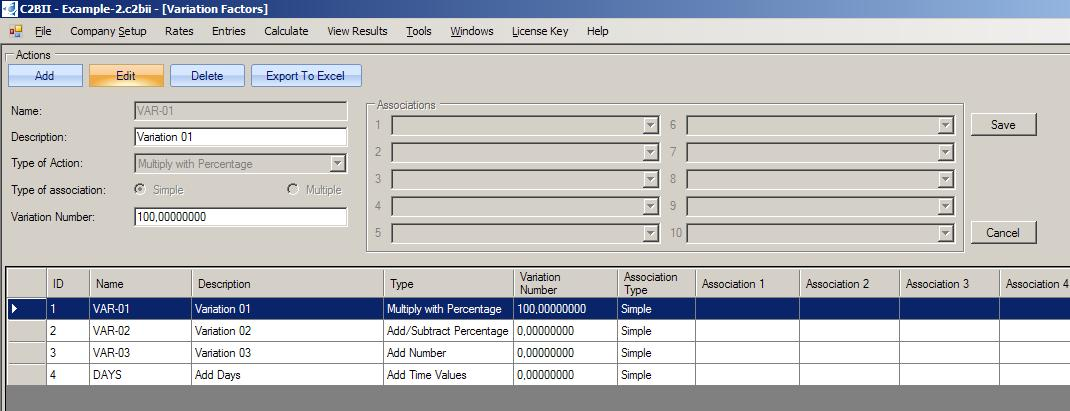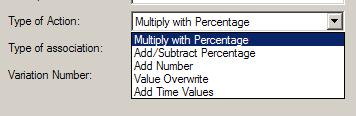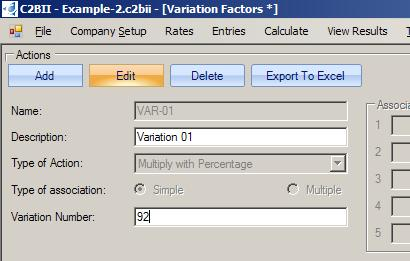# What is the basis of the method of C2BII? Part 7: Processing of “What if” scenarios thru “Variation Factors”

In an “Annual Budget” and in “Investment Plan Evaluation”, the initial work that we have created that calculates the profitability, is simply the starting point for the really important part of the Financial Analysis, which is the processing of “What if” scenarios. Examples of that, might be, what the profitability will look like:

• if we make only 92% of the sales target
• if the days of credit to the customers are 19 more than we anticipated
• if the Interest rates do this
• if the cost of raw materials does the other thing
• if … if … if …

It is important to understand that in the profitability calculation, changes do not come in a linear manner. For example: an increase in sales by 30% does not bring an increase in profitability by the same rate. As a matter of fact, in a specific scenario, it might be possible that it might bring a decrease in profitability by 10%. A huge number of relations exist between the relevant data. In practice, a simple to calculate answer is not feasible. One must recalculate everything from the start.

In C2BII the mechanism that we use to address those alternative scenarios is called “Variation Factors”. Every number that is being entered in the C2BII program (both “set-up data” and “data of forecasted values”) has the capability to be associated with a “Variation Factor”.That association is “non mandatory”. The choice whether it should be utilized at all, and if so, in what manner, is a matter of creative thinking and depends on one’s knowledge of the company’s present practices, and the ability to foresee what might be useful in a novel future situation.A “Variation Factor”, in its most simple form, is the combined impact of two pieces of information. The first is a “Variation Number” and the second is a “Type of Action”.The suggested method (and foreseeable the most common situation) is for “Variation Factors” to be created so that initially they enforce no change to the numerical values they have been associated with. Let us say that an entry with a value of 10,000 is associated with a “Variation Factor”, whose “Type of Action” is “Multiply with Percentage”, and its “Variation Number” is 100. In practice nothing changes, because any number multiplied with 100% remains the same. However, if one wishes to turn that number into 92% of its original value, then the “Variation Number” should be changed into 92. By that, during the calculation process, the original value of 10,000 will turn into 9,200. Every value that is a product of a calculation (examples: “Payment of VAT”, “Interest Income”, “Interest Expense”, “Income Tax” etc) will be calculated again with the new forecasted numbers. Then, all that the user has to do, is to examine the difference between the new and the old profitability results.Stick around, as we are going to see the perils of thinking that we can ignore aspects of the calculation process, and thru abstraction, focus our attention only on the big numbers and the big issues.

This entry was posted in Budget, Budgeting, C2BII Instructions, CashFlow, Financial Analysis, Financial Analysis Method, Investment Plan Evaluation and tagged , , , , , , . Bookmark the permalink.